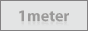# Converters

Torque ( 1 DaN/m = 10 N/m )
 From: To: newton meter [N*m] kilonewton meter [kN*m] kilogram-force meter ounce-force foot [ozf*ft] ounce-force inch [ozf*in] pound-force foot [lbf*ft] pound-force inch [lbf*in] newton meter [N*m] kilonewton meter [kN*m] kilogram-force meter ounce-force foot [ozf*ft] ounce-force inch [ozf*in] pound-force foot [lbf*ft] pound-force inch [lbf*in] Result:
Force
 From: To: newton [N] dekanewton [daN] kilogram-force [kgf] pound-force [lbf] ounce-force [ozf] newton [N] dekanewton [daN] kilogram-force [kgf] pound-force [lbf] ounce-force [ozf] Result:
Volume
 From: To: cubic meter [m^3]cubic kilometer [km^3]cubic decimeter [dm^3]cubic centimeter [cm^3, cc]cubic millimeter [mm^3]liter [L, l]exaliter [EL]petaliter [PL]teraliter [TL]gigaliter [GL]megaliter [ML]kiloliter [kL]hectoliter [hL]dekaliter [daL]deciliter [dL]centiliter [cL]milliliter [mL]microliter [µL]nanoliter [nL]picoliter [pL]femtoliter [fL]attoliter [aL]cc [cc, cm^3]dropbarrel (oil) [bbl (oil)]barrel (US) [bbl (US), bbl liq (US)]barrel (UK) [bbl (UK)]gallon (US) [gal (US)]gallon (UK) [gal (UK)]quart (US) [qt (US), qt liq (US)]quart (UK) [qt (UK)]pint (US) [pt (US), pt liq (US)]pint (UK) [pt (UK)]cup (US)cup (metric)cup (UK)fluid ounce (US) [fl oz (US)]fluid ounce (UK) [fl oz (UK)]tablespoon (US)tablespoon (metric)tablespoon (UK)dessertspoon (US)dessertspoon (UK)teaspoon (US)teaspoon (metric)teaspoon (UK)gill (US) [gi]gill (UK) [gi (UK)]minim (US)minim (UK)cubic mile [mi^3]cubic yard [yd^3]cubic foot [ft^3]cubic inch [in^3]ton register [ton reg]ccfhundred-cubic footacre-foot [ac*ft]acre-foot (US survey) [ac*ft]acre-inch [ac*in]dekasterestere [st]decisterecord [cd]tunhogsheadboard footdram [dr]cor (Biblical)homer (Biblical)bath (Biblical)hin (Biblical)cab (Biblical)log (Biblical)Taza (Spanish)Earth's volume cubic meter [m^3]cubic kilometer [km^3]cubic decimeter [dm^3]cubic centimeter [cm^3, cc]cubic millimeter [mm^3]liter [L, l]exaliter [EL]petaliter [PL]teraliter [TL]gigaliter [GL]megaliter [ML]kiloliter [kL]hectoliter [hL]dekaliter [daL]deciliter [dL]centiliter [cL]milliliter [mL]microliter [µL]nanoliter [nL]picoliter [pL]femtoliter [fL]attoliter [aL]cc [cc, cm^3]dropbarrel (oil) [bbl (oil)]barrel (US) [bbl (US), bbl liq (US)]barrel (UK) [bbl (UK)]gallon (US) [gal (US)]gallon (UK) [gal (UK)]quart (US) [qt (US), qt liq (US)]quart (UK) [qt (UK)]pint (US) [pt (US), pt liq (US)]pint (UK) [pt (UK)]cup (US)cup (metric)cup (UK)fluid ounce (US) [fl oz (US)]fluid ounce (UK) [fl oz (UK)]tablespoon (US)tablespoon (metric)tablespoon (UK)dessertspoon (US)dessertspoon (UK)teaspoon (US)teaspoon (metric)teaspoon (UK)gill (US) [gi]gill (UK) [gi (UK)]minim (US)minim (UK)cubic mile [mi^3]cubic yard [yd^3]cubic foot [ft^3]cubic inch [in^3]ton register [ton reg]ccfhundred-cubic footacre-foot [ac*ft]acre-foot (US survey) [ac*ft]acre-inch [ac*in]dekasterestere [st]decisterecord [cd]tunhogsheadboard footdram [dr]cor (Biblical)homer (Biblical)bath (Biblical)hin (Biblical)cab (Biblical)log (Biblical)Taza (Spanish)Earth's volume Result:
Length
 From: To: foot [ft] foot (US survey) [ft] inch (US survey) [in] inch [in] meter [m] kilometer [km] hectometer [hm] dekameter [dam] decimeter [dm] centimeter [cm] millimeter [mm] light year [ly] league [lea] nautical league (UK) nautical league (int.) league (statute) [st.league] mile [mi, mi(Int)] nautical mile (UK) [NM (UK)] nautical mile (international) mile (statute) [mi, mi (US)] mile (US survey) [mi] yard [yd] foot [ft] foot (US survey) [ft] inch (US survey) [in] inch [in] meter [m] kilometer [km] hectometer [hm] dekameter [dam] decimeter [dm] centimeter [cm] millimeter [mm] light year [ly] league [lea] nautical league (UK) nautical league (int.) league (statute) [st.league] mile [mi, mi(Int)] nautical mile (UK) [NM (UK)] nautical mile (international) mile (statute) [mi, mi (US)] mile (US survey) [mi] yard [yd] Result:
Pressure
 From: To: psi [psi]barmillibar [mbar]pascal [Pa]hectopascal [hPa]newton/square centimeternewton/square millimeterdyne/square centimeterkilogram-force/square meterkilogram-force/square centimeterkilogram-force/square millimetergram-force/square centimeterton-force (short)/square footton-force (short)/square inchton-force (long)/square footton-force (long)/square inchkip-force/square inch [kipf/in^2]ksi [ksi]pound-force/square footpound-force/square inchpoundal/square foot [pdl/ft^2]torr [Torr]centimeter mercury (0°C) [cmHg]millimeter mercury (0°C) [mmHg]inch mercury (32°F) [inHg]inch mercury (60°F) [inHg]centimeter water (4°C) [cmAq]millimeter water (4°C) [mmAq]inch water (4°C) [inAq]foot water (4°C) [ftAq]inch water (60°F) [inAq]foot water (60°F) [ftAq]atmosphere technical [at]Standard atmosphere [atm] psi [psi]barmillibar [mbar]pascal [Pa]hectopascal [hPa]newton/square centimeternewton/square millimeterdyne/square centimeterkilogram-force/square meterkilogram-force/square centimeterkilogram-force/square millimetergram-force/square centimeterton-force (short)/square footton-force (short)/square inchton-force (long)/square footton-force (long)/square inchkip-force/square inch [kipf/in^2]ksi [ksi]pound-force/square footpound-force/square inchpoundal/square foot [pdl/ft^2]torr [Torr]centimeter mercury (0°C) [cmHg]millimeter mercury (0°C) [mmHg]inch mercury (32°F) [inHg]inch mercury (60°F) [inHg]centimeter water (4°C) [cmAq]millimeter water (4°C) [mmAq]inch water (4°C) [inAq]foot water (4°C) [ftAq]inch water (60°F) [inAq]foot water (60°F) [ftAq]atmosphere technical [at]Standard atmosphere [atm] Result:
Temperature
 From: To: kelvin [K] degree Celsius [°C] degree Fahrenheit [°F] Triple point of water kelvin [K] degree Celsius [°C] degree Fahrenheit [°F] Triple point of water Result: